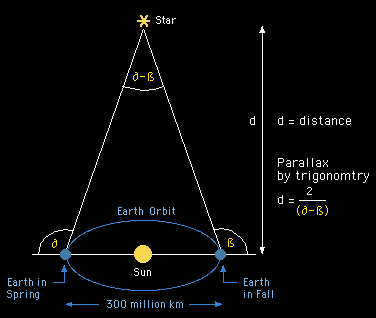1. I was just wondering how i could find the distance between earth and an object in space, i have heard of parallax formula but i am not quite positive on how it works.
Any help or explanation would be greatly appreciated!2.

3. Measuring the distance of a star via the parallax is actually triangulation.
It goes a bit like this:Yet, CalSpace reports that this method only works when the stars are <500 ly away.

This video of the Khan Academy explains how this works:
Parallax in Observing Stars - YouTube

Source:

Methods of Observational Astronomy4. Thanks for the help it was very useful5.Originally Posted by LlamaspaceballThanks for the help it was very useful

You are welcome.6.7.Originally Posted by artiyousharmaits someting formula like c= mc2 hope its help you where c is velocity of time
What?
Your "equation" is meaningless with "c" on both sides of the equation.
Even if you meant e=mc2 that has nothing to do with the topic as such.
And, to top it off, c is the speed of light and time doesn't have a "velocity".8. It's supposed to be d=mc2 where 'd' is the velocity of distance.9. Not my equation, no...

-'m' is Mustard
-'c' is Cheese.
Since c is squared, you have another 'c' which is Cut.
But here's a tip: Finding the square root won't cut it.10.Originally Posted by NeverflyIt's supposed to be d=mc2 where 'd' is the velocity of distance.Shouldn't we factor in the length of height and the weight of red?11.Originally Posted by DywyddyrOriginally Posted by NeverflyIt's supposed to be d=mc2 where 'd' is the velocity of distance.Shouldn't we factor in the length of height and the weight of red?
Only if calculating it in in a Lagrangian Metric with more than one Hamiltonian for the system.distance, formula, parallax, space